## Setup time

There are two types of setup time. One is the time of setup task before the production task, the other one is the time of teardown task after the production task.

Setup time

The setup time is determined by the following settings.

 A1 Static setup “Setup” property of integrated master table Defines the setup time to start the manufacturing task for that operation. A2 “Setup” property of resource table Defines the setup time to start the manufacturing task for that operation. B1 Dynamical setup (changeover) Item setup table Defines the setup time according to the combination of main products of the left operation and the right operation. This is the item switching setup. B2 Resource setup table Defines the setup time according to the combination of the resources of the left operation and the right operation. This is the setup time required for jig switching, the change of workers, and so on. B3 Spec n setup table (n=1, 2, 3, 4) Defines the setup time according to the combination of the specifications of the left operation and the right operation. C1 Overwrite User specified setup time Overwrites the previous setup time calculated from A1, A2, B1, B2, B3. C2 EIIFilterCalcDuration of COM IF Overwrites the previous setup time calculated from A1, A2, C1. C3 EIIFilterCalcCombinationSetupDuration of COM IF Overwrites the setup time calculated from B1, B2, B3. C4 “Zero setup time between same items” project setting If the main product are the same on the main resource, makes the setup time calculated from A1, A2, B1, B2, B3 zero. C5 “Zero setup time between same split operations” project setting If the split operation root is the same for the main resource, makes the setup time calculated from A1, A2, B1, B2, B3 zero.

B1, B2, B3 are tables that define the setup time for each combination of adjacent operations in a resource time series. Such setup time is called dynamical setup time or changeover time.
On the other hand, A1 and A2 define setup times that do not depend on adjacent work, so this is called static setup time.
C1, C2, C3 overwrite the setup time defined in A1, A2, B1, B2, B3.

Teardown time

The teardown time is determined by the following settings.

 X1 Static setup “Teardown” property of integrated master table Defines the setup time after the manufacturing task for that operation has been completed. X2 “Teardown” property of resource table Defines the setup time after the manufacturing task for that operation has been completed. Y1 Overwrite User specified teardown time Overwrites the teardown time calculated from X1, X2. Y2 EIIFilterCalcDuration of COM IF Overwrites the teardown time calculated from X1, X2, Y1. Y3 “Zero setup time between same items” project setting If the main product are the same on the main resource, makes the setup time calculated from X1, X2 zero. Y4 “Zero setup time between same split operations” project setting If the split operation root is the same for the main resource, makes the setup time calculated from X1, X2 zero.

Setup time calculation method

You can choose whether to set up the setup time as the sum of the setup times defined by A1, A2, B1, B2, B3, or as the maximum value.
This is specified in “Setup time calculation method” in the project settings.

 Calculation method Expression Meaning 1 Max value Max( (A1 or A2), B1, B2, B3) Maximum value of static setup that was found first and all dynamical setups 2 Total value (A1 or A2) + B1 + B2 + B3 Total value of static setup that was found first and all dynamical setups 3 Max value(Only one dynamic setup) Max( (A1 or A2), (B1 or B2 or B3) ) Maximum value of static setup that was found first and dynamical setup that was found first 4 Total value(Only one dynamic setup) (A1 or A2) + (B1 or B2 or B3) Total value of static setup that was found first and dynamical setup that was found first

A1 or A2 means searching the settings in the order A1, A2, and applying the first one found.
1 and 2 are new functions of Ver. 9.0.4.0, and a restricted feature of Ver. 10.

For example, suppose that the settings are as follows.

Integrated Master table

 Item Process number Process code Instruction code Setup Production Teardown A 10 P1 M 10

Resource table

 Code Setup Production Teardown R 5 1mp 10

Item setup table

 Resource code Item L Item R Setup time R * * 30M

Resource setup table

 Resource code Resource L Resource R Setup Time R * * 60M

Spec1 setup table

 Resource code Previous spec Previous spec Setup time R * * 90M

Let’s suppose that operations OP1 and OP2 assign resources to R, S1 and S2 as follows.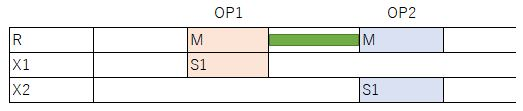In this case, the setup time according to the method of calculating the setup time of operation OP2 is as follows.

 Calculation method Expression Result Max value Max( (A1 or A2), B1, B2, B3) Max (10 minutes, 30 minutes, 60 minutes, 90 minutes) = 90 minutes Total value (A1 or A2) + B1 + B2 + B3 10 minutes + 30 minutes + 60 minutes + 90 minutes = 190 minutes Max value(Only one dynamic setup) Max( (A1 or A2), (B1 or B2 or B3) ) Max (10 minutes, 30 minutes) = 30 minutes Total value(Only one dynamic setup) (A1 or A2) + (B1 or B2 or B3) 10 minutes + 30 minutes = 40 minutes

For example, if the preparation time that always occurs when using that resource is set to “Setup” of the Integrated Master Editor table, the process tooling switch time is set to the Resource Setup table, and the time put into equipment when work in progress is switched is set to the Item Setup table, and these three setup operations can be performed in parallel, set “MAX value”, and if cannot be performed in parallel, set “Total value”. Regarding (only one dynamical setup), see the explanation of resource setup below.

From the item setup table

Setup time is calculated by looking up the record in the item setup table corresponding to the resource to which the operations are assigned and the item codes of output instructions of the lefthand and righthand operations.
For example, suppose that the item setup table is specified as follows.

 Resource Item L Item R Setup time Sort order * * * 5 1.0 R1 = = 0 2.0 RG1 IG1 I3;I4 7 3.0 R1;R2 I1 I2 15 4.0 R1;R2 I2 I1 10 5.0 R1 ! I1 10 6.0

*RG1 here presents a resource group, and IG1 represents an item group.

The first record means that "for any resource, from any item to any item, the setup time is 5 minutes." "*" means "any".
The second record means that "the setup time on resource R1 is 0 between identical items".
"=" means "the same as the other item"; if both items are marked "=", it means "when the previous and following items are the same".
The third record means that "for any resource in resource group RG1, from any item in item group IG1 to item I3 or I4, the setup time is 7 minutes."
As in the third, fourth, and fifth records, you can specify groups and multiple values in the Resource, Item L, and Item R fields.
When "!" is specified in the Item L field as in the sixth record, it means that there is no operation on the left side. For example, the leftmost operations on the resource Gantt chart.
Note that if we are looking up the setup time for operations on resource R1 from I1 to I2, the answer is not uniquely defined, since our conditions are matched by both record 1 and record 4.
For this reason, we specify a sort order, so that the records will be searched beginning with the highest sort order, using the first record that matches.
In general, you should give small sort orders to records containing general information, for example when specifying default settings.Higher sort orders should be assigned to records containing more specific information, as when specifying settings relating to particular items.

In addition, the "!=" mark is available from Ver.9.0.4.0 (Ver.10 restricted feature). It means the item of left operation is different with the item of right operation. The setup time setting of R3 is the same with the onf of R4 as follows.

 Resource Item L Item R Setup time Sort order R3 * * 7 1.0 R3 = = 0 2.0 R4 != != 7 1.0

The marks ( *, =, !=, !) as follows can be used in the "Item L" and "Item R" columns. The combinations except the four combinations as follows are ignored.

 Mark Combination * *, item group, item, ! ! *, item group, item = = != !=

In addition, in the case that the "Setup time factor" property of resource is specified, the resource changeover setup time will be effected.

The setting method is aslo available for other changeover setup time table.

From the spec n setup table (n=1, 2, 3, 4)

Setup time is calculated by looking up the record in the spec n setup table corresponding to the resource to which the operations are assigned and the spec n of output instructions of the lefthand and righthand operations.
Otherwise the method is the same as for the item setup table.

From the resource setup table

You can define setup time for main resource and sub resource.It is used to define the setup time when the main resource of left operation is different with the main resource of right operation.

By making the following settings in the resource setup table,

 Resource Resource L Resource R Setup Time Sort order R X1 X2 10

the setup time (10 minutes) occurs when the sub resource switches from X1 to X2 when moving from operation OP1 to OP2, as follows.Resource setup time by instruction code (Ver. 15 restricted feature)

Normally, one object from each changeover table is selected, but if one specifies an instruction code and specifies a setup time, one can select multiple resource setup objects. In the following example, a resource setup is defined for instruction code S1 and instruction code S2.

For example, if S1 is a mold and S2 is an operator instruction, and you want to total the time required for switching between the mold and the operator, this is defined as follows.

 Resource Instruction code Resource L Resource R Setup Time Sort order R S1 X1 X2 10 R S2 Y1 Y2 20

If operations OP1 and OP2 are assigned as shown in the figure below, the setup time occurs as follows.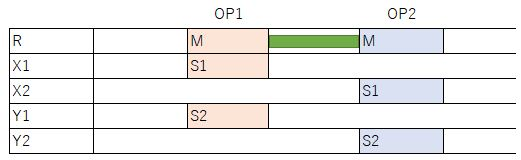At this time, the setup time by instruction code S1 (mold switching) and S2 (operator change) is as follows.

 Method of calculating setup time in project settings Expression “Total value” or “Total value (only 1 dynamical setup)” 10 minutes + 20 minutes = 30 minutes “Max value” or “Max value (only one dynamical setup)” Max (10 minutes, 20 minutes) = 20 minutes

This may be easier to understand if you think that a resource setup table is newly created for each instruction code in the resource setup table.
In other words, this is as follows.

 Method of calculating setup time in project settings Expression “Total value” or “Total value (only 1 dynamical setup)” Static setup + item setup + specification 1 setup ... + specification n setup + resource setup (S1) + resource setup (S2) “Max value” or “Max value (only one dynamical setup)” Max (Static setup, item setup, specification 1 setup ..., specification n setup, resource setup (S1), resource setup (S2))

Here is another example, where an instruction code should be used.

For example, suppose that the following settings are made, without specifying an instruction code. The intent is to have a setup time occur when resource switching occurs.

 Resource Instruction code Resource L Resource R Setup Time Sort order R != != 10

However, with these settings, when operations OP1 and OP2 are assigned as follows, looking at each instruction code, resources are not switched, but when looked at from the viewpoint of resource R, switching from X1 to Y1 and from Y1 to X1 is considered to have occurred, and a setup time will occur.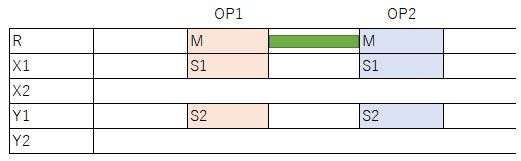If you specify an instruction code as follows, no setup time will occur.

 Resource Instruction code Resource L Resource R Setup Time Sort order R S1 != != 10 R S2 != != 10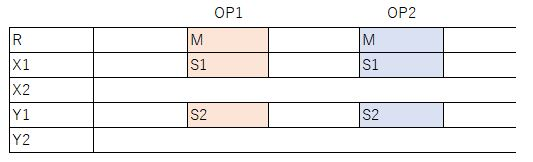Further, even if you specify multiple instruction codes with semicolon separators in "Instruction code" as follows, or specify “*”, no setup time will occur.

 Resource Instruction code Resource L Resource R Setup Time Sort order R S1;S2 != != 10

 Resource Instruction code Resource L Resource R Setup Time Sort order R * != != 10

Sample data is available. For further details please see the Sample A-2 project file.

"Resource setup time selection method” (specification change tab) in project settings

* This property is for maintaining compatibility with the specifications of past versions. Basically, set "Sort order" or specify the "Instruction code" property to define the setup time for each instruction code.

In the above example, next let’s consider the case of not having specified an instruction code this time.
At this time, only one resource setup object must be selected from the resource setup table.

 Resource Instruction code Resource L Resource R Setup Time Sort order R X1 X2 10 1 R Y1 Y2 20 2

 "Resource setup time selection method” in project settings Sort order In the resource setup table, search all the resource setup objects to which the "resource" property is applicable, and select the one with the highest sort order. ? 20 minutes Order of appearance In the resource setup table, search the resource setup objects to which the "resource" property is applicable, and select the one that is found first. ? 10 minutes

"Resource setup time selection method" property is only can be used from Ver.8.0.2. In case of before Ver.8.0.2, "Order of appearance" is selected.

"Max value (only one dynamical setup)” "Total value (only one dynamical setup)” in “Setup time calculation method” in the project settings

* The Enum of this property is for maintaining compatibility with the specifications of past versions. If you have a license of Ver. 10 or higher, set it either to "Maximum value" or "Total value", or specify the setup time for each instruction code by specifying the "Instruction code" property.

This is set as either checking resource switching between adjacent operations with all use instructions, or checking from the main resource in order, and if not found, ending.

For example, suppose that the resource setup table is specified as follows.

 Resource Instruction code Resource L Resource R Setup Time Sort order X R1 R2 10 1 Y R1 R2 30 2

Furthermore, it is assumed that the setup time was defined with instruction codes S1 and S2 in the Integrated Master.
In this case, if operations OP1 and OP2 are assigned, a setup time will be generated in OP2.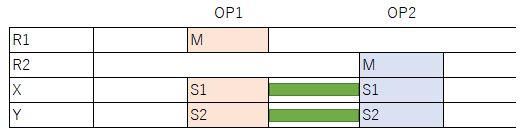Although the above two resource setup objects correspond to the setup time, one resource setup object must be selected from the resource setup table because no instruction code is specified.

 “Setup time calculation method” in Project settings "Total value" or "Maximum value” Select the one with the highest sort order of all applicable resource setup objects. ? 30 minutes “Total value (only 1 dynamical setup)” or “Maximum value (only 1 dynamical setup)” Acquires the resources of the use instructions in ascending order of sibling numbers, and searches the table for the applicable resource setup objects for that resource. In the example of the figure above, resource setup objects are searched in the order of M ? S1 ? S2. ? 10 minutes

Difference between “specifying !=” and “specifying * and =”

If set as follows, if even one resource switching occurs, setup time will be generated.

 Resource Instruction code Resource L Resource R Setup Time Sort order * != != 10 1In a similar setting, if the sorting order is specified as follows, even if you set the “Setup time calculation method” in the project settings to “Maximum value” or “Total value” and calculate the setup time for all use instructions, if the same resources are consecutive in one use instruction, the resource setup object of the setup time of the first row = 0 seconds is selected, and no setup time occurs.

 Resource Instruction code Resource L Resource R Setup Time Sort order * = = 0 2 * * * 10 1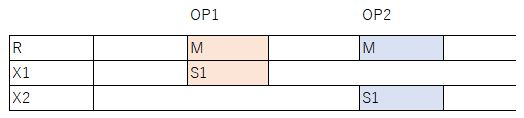Therefore, in such a case, set !=.

External Setup Time

An external setup time is assigned to a different resource from the resource to which the use instruction of the production task is assigned. An external setup time is assigned to a different resource from the resource to which the use instruction of the production task is assigned.

You can specify external setup as follows:

Integrated master editor
 Item Process number Process code Instruction type Instruction code Resource/item Setup Production ItemA 10 Pr1 Use instruction M Equipment1 10mp ItemA 10 Pr1 Use instruction I External setup equipment 60m

In the example above, the external setup time for Equipment1 is 60 minutes and it is assigned to the External setup equipment.

Link to Changeover Object from Operation

Operations contain link information to applicable changeover objects, which you may confirm in the link tab of the properties window.
As item setup applies to the following operation, the information is specified in "Changeover object (item setup)."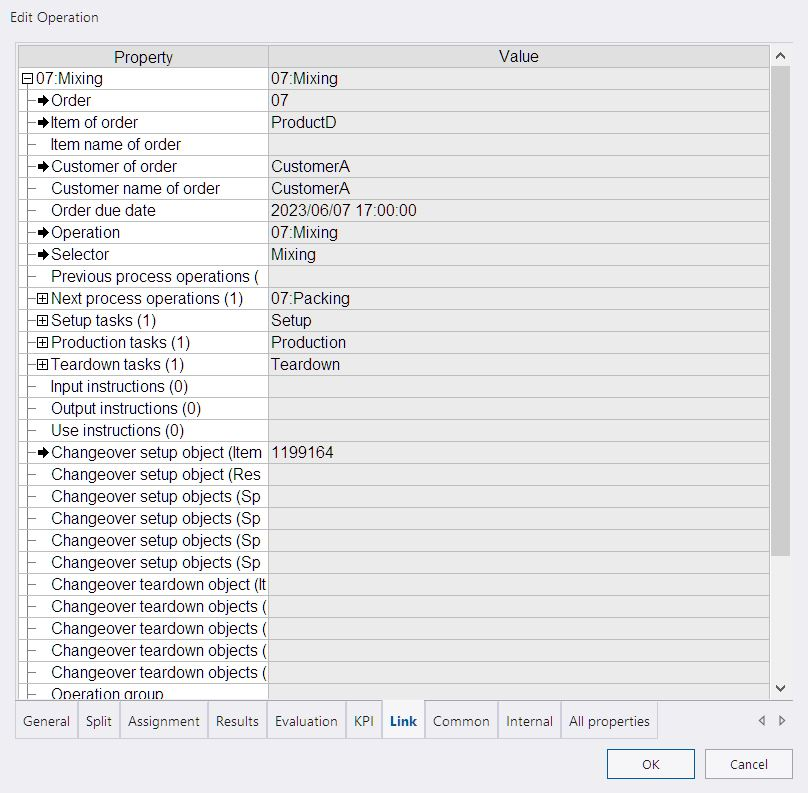You may jump to the changeover object by clicking the black arrow in "Changeover object (item setup)."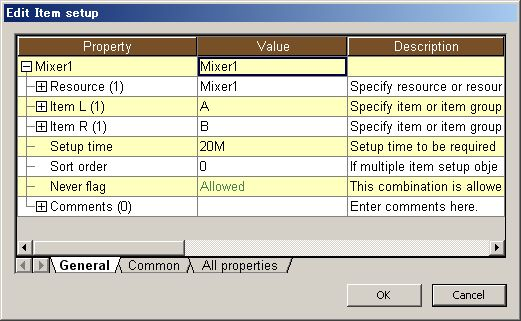* The actual setup time is dependent on the setup time calculation method in addition to the linked changeover object.